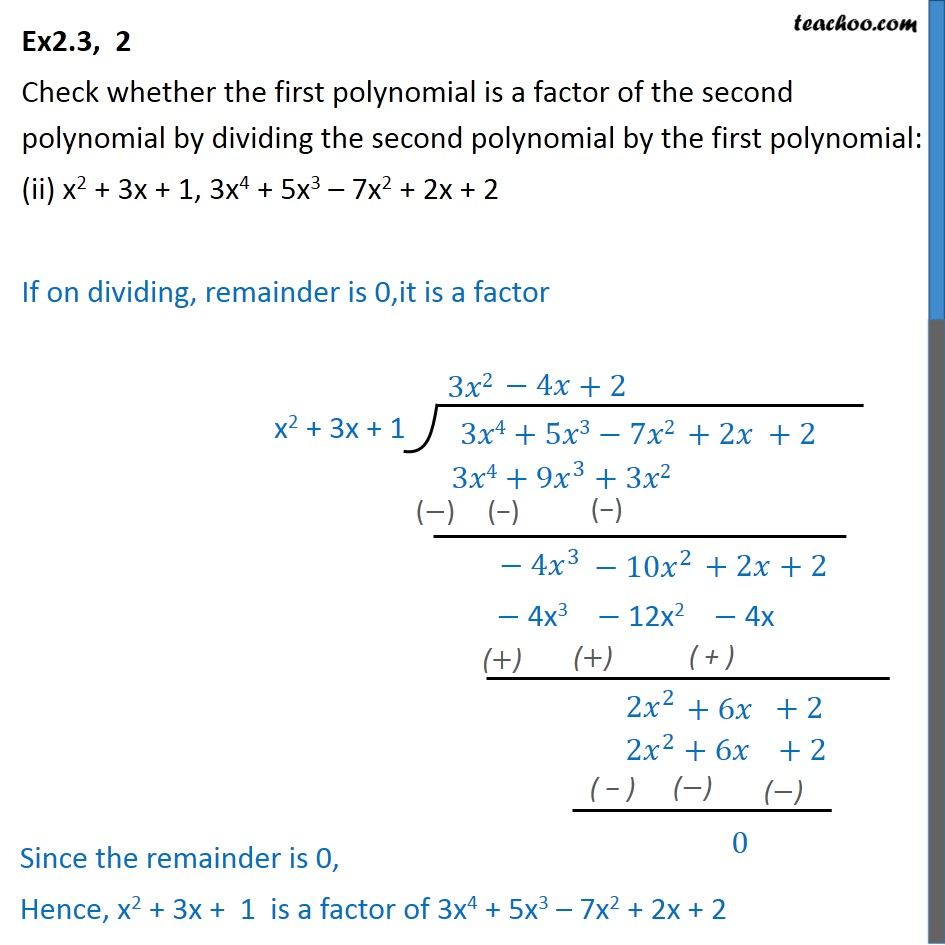Division Algorithm

Chapter 2 Class 10 Polynomials
Serial order wiseLearn in your speed, with individual attention - Teachoo Maths 1-on-1 Class

### Transcript

Ex2.3, 2 Check whether the first polynomial is a factor of the second polynomial by dividing the second polynomial by the first polynomial: (ii) x2 + 3x + 1, 3x4 + 5x3 7x2 + 2x + 2 If on dividing, remainder is 0,it is a factor